# Use the results of Prob. 7-18 and let the unperturbed guide be loss-free, so that In the perturbed guide, let And show that If Z = the intrinsic impedance of metal walls, the above formula for a is the approximation that we have been using to calculate attenuation in metal waveguides. Prob. 7-18 Consider the perturbation of the walls of a waveguide from a perfect conductor to an impedance sheet Z such that Represent the unperturbed and perturbed fields as in Prob. 7-15, and show that Prob. 7-15 Suppose that a waveguide is filled with lossy material, and consider a perturbation of its perfectly conducting walls. Represent the unperturbed fields (subscript 0) and the perturbed fields (no subscript) by Note the opposite directions of propagation. Show that the formula corresponding to Eq. (7-29) is Show that this reduces to Eq. (7-29) in the loss-free case.

Question
1 views

Use the results of Prob. 7-18 and let the unperturbed guide be loss-free, so that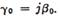In the perturbed guide, let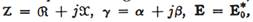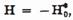And show that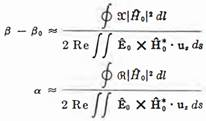If Z = the intrinsic impedance of metal walls, the above formula for a is the approximation that we have been using to calculate attenuation in metal waveguides.

Prob. 7-18

Consider the perturbation of the walls of a waveguide from a perfect conductor to an impedance sheet Z such that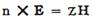Represent the unperturbed and perturbed fields as in Prob. 7-15, and show that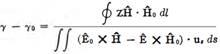Prob. 7-15

Suppose that a waveguide is filled with lossy material, and consider a perturbation of its perfectly conducting walls. Represent the unperturbed fields (subscript 0) and the perturbed fields (no subscript) by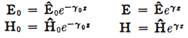Note the opposite directions of propagation. Show that the formula corresponding to Eq. (7-29) is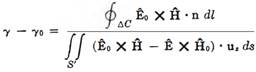Show that this reduces to Eq. (7-29) in the loss-free case.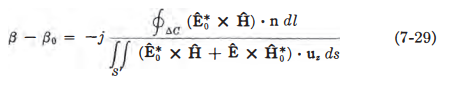### This question hasn't been answered yet.